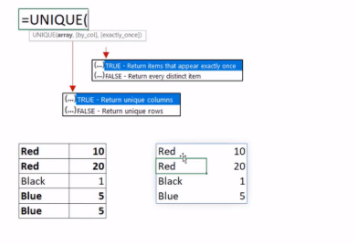# Excel UNIQUE FunctionIn this tutorial, we will learn about An Excel Formula called UNIQUE to Remove Duplicate Values in Excel

Here I have a table of 3 different colors named as Red, Blue and, Black. Now I want to remove the duplicate items from the list such that all 3 colors should be unique in the list.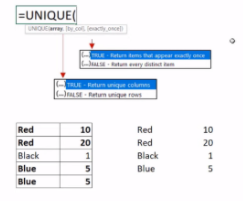So to remove the duplicate items from the list, we will be applying a UNIQUE Formula.

1. Write a UNIQUE function

2. Choose the range of values where 3 colors are mentioned and close the bracket =UNIQUE(B14:B18)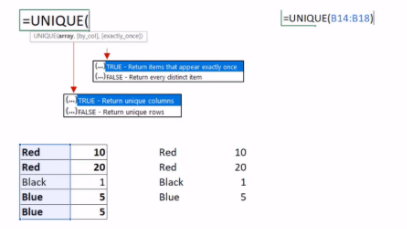3. Press Enter, Now you will see the duplicate items have been removed from the list and unique items has been placed in the list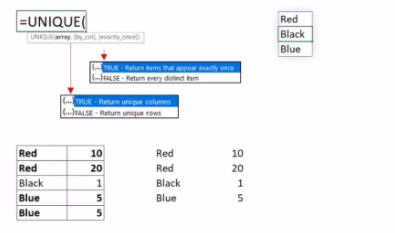How to Remove Duplicate Values in a given row?

In this table, according to color-wise, there are 3 different colors which are Red, Black, and Blue. Now there are 2 things inside the table which are different

1. In Red Color there are 2 different entries denominated as 10 and 20 according to Row wise

2. In Blue Color there are common entries denominated as 5 and 5 according to Row wise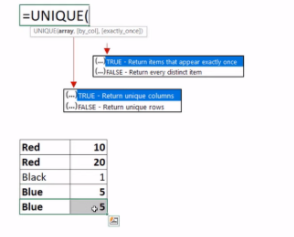So here we will remove all duplicates values in a given row by applying Unique Formula

1. Write a UNIQUE function

Now here there are 3 parameters (array, [by_col], [exactly_once]).
The first parameter array means selecting the entire table

2. Select the entire table =UNIQUE(B14:C18,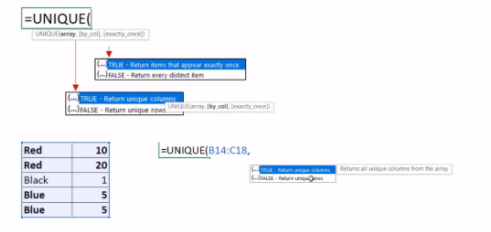The second parameter [by_col] means to compare and extract. By row = FALSE By column = TRUE.

3. Press FALSE as Return unique rows =UNIQUE(B14:C18, FALSE,

The third parameter [exactly_once] means TRUE = values that occur once, FALSE= all unique values

4. Press FALSE as Return distinct items and close the bracket =UNIQUE(B14:C18, FALSE, FALSE)

5. Press Enter

6. Lastly you will see that the duplicate items have been removed from the given table